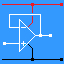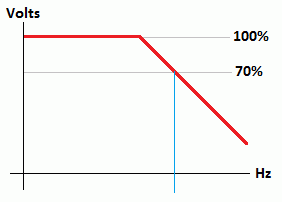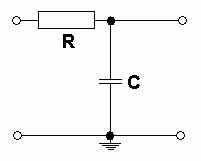RANDOM PAGE

SITE SEARCH

LOG
IN

HELP

# Passive Filters

This is the AQA version closing after June 2019. Visit the the version for Eduqas instead.

## Filters

Filters are used to pass or block selected ranges of frequencies.

• Sub-woofer: this uses a low pass filter to send the bass to the sub-woofer. The mid range and high frequencies are removed.
• Tweeter: this uses a high pass filter to send the treble audio to the tweeter. The mid range and bass are removed.
• Low Pass Filters are important when converting analogue signals to digital. If frequencies greater than half the sampling rate get to the converter, unwanted and unpleasant alias signals appear at the output.

## Filter Breakpoint Frequency

At the breakpoint frequency of a filter ...

• the output voltage drops to 0.7 of the maximum output voltage.
• the reactance of the capacitor is equal to the resistance of the resistor.

## Passive RC Filters

• In the graphs below, the blue vertical lines show the breakpoint frequencies.
• Both the X and Y graph axes are log scales.
• Passive filters attenuate the signals. If this is a problem, active filters should be used instead.

### High Pass - Bass Cut• The capacitor blocks DC and low frequencies.
• The capacitor passes high frequencies easily.

### Low Pass - Treble Cut• The capacitor has no effect on DC or low frequencies.
• The capacitor decouples high frequencies to ground.

### Band Pass• This circuit is a combination of
high and low pass circuits

### Capacitor Reactance

• Reactance is the Alternating Current equivalent of resistance.
• It is measured in Ohms.
• The formula: Xc = 1 / (2 π f C)

### Breakpoint Frequency Calculations

• These calculations apply to both passive and active filters.
• At the breakpoint frequency, the reactance of the capacitor equals the resistance of the resistor.

• R = Xc

• Xc = 1 / (2 π f C)

• R = 1 / (2 π f C)

• R f = 1 / (2 π C)

• f = 1 / (2 π R C)

• C = 1 / (2 π R f)

reviseOmatic V3     Contacts, ©, Cookies, Data Protection and Disclaimers Hosted at linode.com, London